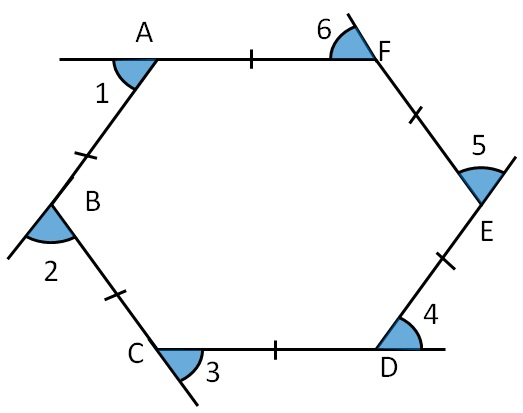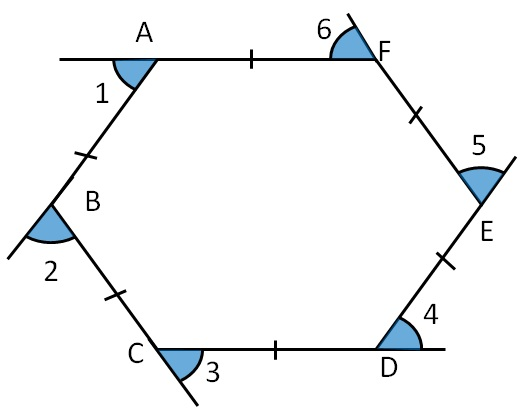Sum of Exterior Angles of PolygonsHere,

∠1, ∠2, ∠3, ∠4, ∠5 and ∠6 are the exterior angles of Regular Polygon.

All exterior angles of a Regular Polygon are equal.

∴ ∠1 = ∠2 = ∠3 = ∠4 = ∠5 = ∠6.

Let’s find the value of exterior angles

### Value of exterior angles

All exterior angles of a regular Polygon  are equal.

∴ ∠1 = ∠2 = ∠3 = ∠4 = ∠5 = ∠6 = x

We know that

Sum of all exterior angles = 360°

x + x + x + x + x + x = 360°

6x  = 360°

x = (360°)/6If number of sides are n ,

Exterior angle = (360°)/n

where n is the number of sides of regular polygon

Get live Maths 1-on-1 Classs - Class 6 to 12